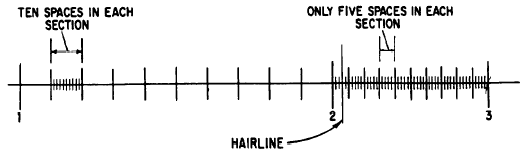Custom SearchOPERATIONS WITH THE SLIDE RULE There are two parts in solving problems with a slide rule. In the first part the slide rule is used to find the digit sequence of the final result. The second part is concerned with the placing of the decimal point in the result. Let us consider first the digit sequence in multiplication and division. Multiplication Multiplication is performed on the C and D scales of the slide rule. The following procedure is used:Figure 8-6.-Reading in the second division of a slide rule. 1. Locate one of the factors to be multiplied on the D scale, disregarding the decimal point.  2. Place the index of the C scale opposite that number. 3. Locate the other factor on the C scale and move the hairline of the indicator to cover this factor. 4. The .product is on the D scale under the hairline. Sometimes in multiplying numbers, such as 25 x 6, the number on the C scale extends to the right of the stock and the product cannot be read. In such a case, we simply shift indices. Instead of the left-hand index of the C scale, the right-hand index is placed opposite the factor on the D scale. The rest of the problem remains the same. By shifting indices, we are simply multiplying or dividing by 10, but this plays no part in reading the significant digits. Shifting indices affects the characteristic only. EXAMPLE: 252 x 3 = 756 1. Place the left index of the C scale over 252. 2. Locate 3 on the C scale and set the hair- line of the indicator over it. 3. Under the hairline on the D scale read the product, 756. EXAMPLE: 4 x 64 = 256 1. Place the right index of the C scale over 4. 2. Locate 64 on the C scale and set the hairline of the indicator over it. 3. Under the hairline on the D scale read the product, 256. Practice problems. Determine the following products by slide rule to three significant digits : 1. 2.8 x 16  2. 7 x 1.3  3. 6 x 85 4. 2.56 x 3.5 Answers: 1. 44.8  2. 9.10  3. 510 4. 8.96 Division Division being the inverse of multiplication, the process of multiplication is reversed to perform division on a slide rule. We subtract the length representing the logarithm of the divisor from the length representing the logarithm of the dividend to get the logarithm of the quotient. The procedure is as follows: 1. Locate the dividend on the D scale and place the hairline of the indicator over it. 2. Move the slide until the divisor (on the C scale) lies under the hairline. 3. Read the quotient on the D scale opposite the C scale index. If the divisor is greater numerically than the dividend, the slide will extend to the left. If the divisor is less, the slide will extend to the right. In either case, the quotient is the number on the D scale that lies opposite the C scale index, falling within the limits of the D scale. EXAMPLE : 6 ũ 3 = 2 1. Locate 6 on the D scale and place the hairline of the indicator over it. 2. Move the slide until 3 on the C scale is under the hairline. 3. Opposite the left C scale index, read the quotient, 2, on the D scale. EXAMPLE : 378 ũ 63 = 6 1. Locate 378 on the D scale and move the hairline of the indicator over it. 2. Move the slide to the left until 63 on the C scale is under the hairline. 3. Opposite the right-hand index of the C scale, read the quotient, 6, on the D scale. Practice problems. Determine the following quotients by slide rule. 1. 126 ũ 3 2. 960 ũ 15 3. 142 ũ 71 4. 459 ũ 17 Answers: 1. 42  2. 64  3. 2 4. 27